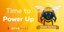# Article 9- Commercial Viability of Alluvial Gold MineThere are factors determining the commercial viability of a gold mine, put simply, whether the gold mine will be profitable at the of the mining operation after calculating the cost the value of the amount of gold mined from the land is more than the overhead cost.
The cost of licking off the overburden is a major cost to consider in this context. If for example, the depth of the overburden is 6 meters and the depth of the AU bearing material is just 1 meter deep, therefore the miner needs to calculate the cost of diesel and labor of licking off the overburden vic and vic the overhead cost minus the cost from the value of gold recovered in the process of recovery.
lets breakdown for the purpose of clarity,
e.g; Diesel cost =\$500
Excavator Operator=\$300
Excavator Hire =\$1000
Salary of 2 staff= \$400
Royalty= \$200
Total gold recovered=200 grams of gold =\$12,390-2400=\$9,990
Therefore you can see now that from the example given, the mining operation we arrive at a gross profit of \$9,990 dollars.
Remember we talked about the Redlines which are regarded by the miner as the richest deposit of the mine and the green line which is where the average of gold is deposited in the gold mine and the yellow line which is where the lowest deposit of gold is found in the mine. Now, let's take for instance, that the data gathered above \$9,990 dollars gross profit is realized from the average line of 2gpt, from 10 tons of AU materials, processed by the miner to realize \$9,990 dollars, it is left for the miner to then decide whether this mine is commercially viable based on data gathered as the net profit.
in addition, you can see that the low line which is the yellow line would certainly not be viable as when you do the math of let's assume, this low line is 1gpt, therefore the math is as follows:
Total Overhead cost of mining =\$2,400
Igpt of 10tons of AU Materials: 10 x 1=10grams of gold
10 grams of gold = \$619.5
Therefore; \$619.5 - \$2400 = -\$1,780.5 Deficit.
from the foregoing, it is clear that the miner need not mine the low line as he will incur a loss, as the line is not commercially viable though there is a gold deposit found on this line and you can now see how much cost and time these data help the miner to save as much.
There are other factors like the carat of the gold, the width, and the total area of the gold deposited should also be put into consideration by the miner to be well informed if the mine is actually commercially viable before embarking on the alluvial gold mining.

Posted Using LeoFinance Beta

0
0
0.000Congratulations @goldmark45! You have completed the following achievement on the Hive blockchain and have been rewarded with new badge(s):You published more than 10 posts.Your next target is to reach 20 posts.

If you no longer want to receive notifications, reply to this comment with the word `STOP`Hive Power Up Day - April 1st 2022